# Performance Measures of Online Warehouse Service System with Replenishment Policy

Performance Measures of Online Warehouse Service System with Replenishment Policy

Samsani Saritha Elliriki MamathaChandra S. Reddy

Department of Mathematics, GITAM University, Bangalore 562163, India

Department of Mathematics, CIT - North Campus, Bangalore 562110, India

Corresponding Author Email:
mamathareddy.elliriki@gitam.edu
Page:
631-638
|
DOI:
https://doi.org/10.18280/jesa.520611
22 July 2019
|
Accepted:
8 October 2019
|
Published:
30 December 2019
| Citation

OPEN ACCESS

Abstract:

In this work, we studied an inventory model with a single-component preservation system and the replenishment schedule as decision variables. The system has characteristics with three distinct states. The productive items are maintained in the warehouse, the items are serviced on demand of the customers, any defective items are to be serviced if it serviceable or discarded. The system is monitored periodical intervals, and the warehouse system is maintained with frequent observations and inspections. Based on the request of the customer the products are serviced. If any defective product is serviced then the customer has the right to the returned product with a new item or amount paid. The defective items that are returned from the customers re-entered into the service system after repair or discarded as scrap. The key intention of the paper is to minimize the optimization of cost, minimal time service, and effective stock of the warehouse. The produced results show that effective improvement with existing metrics. Finally, we formulated an inventory model for the stochastic optimal performance measures of various parameters.

Keywords:

inventory system, replenishment orders, Markov process, queuing system, cost optimization

1. Introduction

Stochastic inventory models are addressed by many researchers in which the ordered product through online sources is not instantaneously delivered to the purchased customers in recent years [1-4]. The stocked items in the warehouse need time to process the product such as order collection, preparation, the package of the item, and dispatch to the customer. The time that takes to deliver a product for the ordered procurer is haphazard and a positive value. It leads to form a queue and inventory executive needs to monitor the waiting time to dispatch the product along with waiting ordered products, inventory size, product holding time and other factors to judge the system performance, to execute different strategies for ordered items [5-7]. An inventory management system handles a single inventory article to service the customer at a time in the service facility. In these papers, authors assumed that service rates, as well as demand, are considered to be deterministic and invariable. The ordered products form a queue for service in the later stage when the stock out in the stockroom [8, 9]. The key focus in these papers is to optimize the total cost rate with the optimal order quantity to generate maximum revenue [10, 11].

Inventory system with a return policy is the system, in which the defective products can be returned from the customers in a repairable state. Demand, service process is independent process and follows an exponential distribution. It is assumed that always demand and supply balanced [12, 13]. So that product is serviced in a stipulated time without extra cost . Regarding the services, it is considered that the customers have the right to return the defective products that are ordered during the warranty time . In this case, another new similar product will be delivered without cost-increasing. The returned products either enter into the service after repair or discarded and treated as scrap.

Yadavalli examined two - product inventory system with general demand and joint ordered product into the service facility [16-18]. The service time to process orders follows exponential distribution where mean service rate always lesser to mean arrival time. The ordered products by the customers require one item at a time to serve in the inventory. For optimal policy under the mean cost of product and discarded item, both bounded and unbound time horizon time is revealed as a threshold in the ordering policy. A relatively logical model was developed by Schwarzet.al. , where the system is a Markovian production system, in which a single server processes the demands as a batch by taking the orders a unit size . Giri and Bardhan  discussed a two-echelon supply chain dependent cost system with partially logging the products.

In this paper, we studied an inventory control system for orders, returns and demands of the products with single-item inventory systems that are processed between warehouse and customers. Inventory control systems with various features such as order of the product, return policy, items repairable and most of these facilities are described in the form of a closed circular queues systems with sufficient size of the products inside the system. Non-feasible defected items that are returned by customers are allowed to discard from the system are addressed in these papers. The decision of disposal products and the validation of all items accepting for returns direct to a highly unpredictable inventory level and consequently leads to sky-scraping inventory price of the products . To scale down the rebuff of the returned item cost, in some cases transshipment of returns is permitted between all inventory systems. Another side of the inventory systems, various models for remanufacturing and resurveying is developed in the papers and feedbacks with compliment reviews along with recent advances associated topics are established. They focused on zero lead times with arbitrarily distributed service times and examined the system with bounded queuing size where orders of customers follow the Poisson process [23-25]. Models have been developed for optimal ordering quantity with a feasible cost structure to optimize long-run predicted cost in a unit cycle time.

The rest of the paper is organized as follows. In section 2 assumptions, nomenclatures and symbols that are commonly used in the development of the inventory system models are discussed. In section 3, an E-commerce sales problem for a single-item inventory model with a return policy is presented. The description of a mathematical model is studied in section 4. The inventory model is further upgraded to the lateral transshipment of returns with a service facility. In section 5, we analyzed the results with optimal performance measures for the remanufacturing inventory system. Finally, in section 6, summary and concluding observations are discussed.

2. Nomenclatures and Assumptions

2.1 Nomenclatures

To develop the mathematical model and performance metrics the following nomenclatures, symbols are used in this manuscript.

Hc - Holding price of the product per quantity in a periodic cycle time

Sc - Shortage price of the product per quantity in a periodic cycle time

Ec - Establishment cost of stock per order

R - Demand price of the product

K -Manufacturing price of the product

t - Scheduling time period

tp - prescribed scheduling time period

z - Stock size

D - Total demand

q - Quantity that exists at the initial stage

2.2 Assumptions

Let the system services the products based on the demand and supply of the inventory model with a single product servicing mechanism. The service process may be in control state or beyond its service state. At the early stage, the system is considered to be an in-control state with non-defective products. If any serviced defective products to the customers may be returned to the service station. The failure products serviced if it is possible otherwise discarded with suitable probability rates. With the following assumptions a mathematical model is constructed:

• The working environment is treated as deterministic.
• The demand rate is proportionate to the service cycle and maybe fewer than the service rate.
• The inventory item's decline is proportional to the invariable fraction and leads to constant in the on-hand inventory system.
• The product purchaser ought to pay handling and shipping charges.
• The deteriorating item cost is constant and vulnerable.
• Shortage if exist is treated as minimal and negligible.
• The elapsed time in the service process is a random variable and follows an exponential distribution.
• The process is inspected at time intervals ti to refer to the state space.

If the system is beyond control, the proposed method allows two possible states:

Case I: The system is restored after optimal service whereas the system failure rate is unaffected.

Case II: If the system is not enough to provide service after the first case then the replenishment is to be ceased.

The service cycle ends its services if the system shifts to the second case or afterfirst case random checkup intervals whichever occurs first. If the system is beyond the service state, bad quality products are revoked without service and defective items that are possible to service enter into service after the repair, rest is discarded

3. Problem Description and Analysis

In this section, we present an inventory cost model that is handled that process item with a continuous surveillance procurement policy and the development of mathematical solutions to find policy parametric. The key, to study state distributions is the usage of normal distribution random variables for net inventory many authors studied at various inventory models of various aspects of managing repairable products. This model mostly assumes failure at the product simultaneously generates the demand for the product, the same type. That is serviceable products and failure product return processes are perfectly correlated.

The main aim of the seller under the present marketing tactic is to promote the products by persuading the customers to purchase the items by giving the facility to return the product if the customer is not satisfied in a stipulated time with the full amount as a refund or with replacement facility. As a result of this policy, offered by the wholesalers, most of the customers may take it as a privilege to purchase the items and they have to tackle all orders and a lot of returns. And most of the cases the returned items may be in fine condition and these items can be reentered into the market for sale by examining items after servicing and repacking. These models are either single – item or multi-items inventory control systems to handle return products is arranged in a queuing network [5, 11, 14]. Demands and returns are two independent processes in the inventory system and pursue the Poisson process. The items returned by customers are reviewed for quality and serviced with standard testing tools. The repaired items entered into the service facility, items failed during the testing time are discarded as scrap. The time to repair products is assumed negligible. The inventory system is a continuous review policy controlled by a well-known (r, Q) model. A schematic view of single item service mechanism system can be observed in Figure 1. The solution of the model is analyzed by using the spectral expansion method to measure various performance measures of the system with steady-state probabilities.

Transshipment of returns in two similar inventory systems from one level of inventory to another for service is presented in an irreducible continuous Markov process in two-dimensional spaces. An analytical method by using a spectral method is given for this model and a robust solution of the system is developed for study state probability distribution. Performance measures of stock movement, average running cost, and time required to process items can be computed for this joint inventory system with the help of fairly accurate probability distribution. Replenishment and inventory costs are the key focus in the system, since there is no loss of demands or accumulated orders where leading time is expected to be negligible or zero during order replenishment [17, 18]. The refusal rate of returned items is tremendously little and considerably declines in the transshipment inventory model when the size of re-ordering items (Q+1) become gigantic. A further model can be upgraded to an inventory system with multiple orders and returns with a single service warehouse station. It is particularly important when remanufacturer contains multiple recycling service places so that all locations can be interconnected with a fair network to exchange information. It leads to a great extent to the rate of clearance products.

## 1.png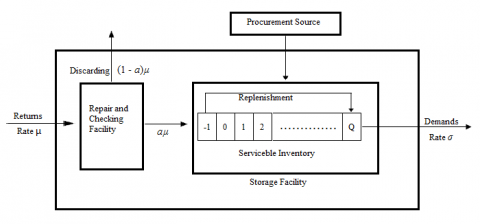Figure 1. A schematic representation of the single – product inventory model

Do not begin a new section directly at the bottom of the page, instead, move the heading to the top of the next page.

The maximum inventory capacity in the system is Q with mean arrival rates σ and service rate µ respectively and follow Poisson processes. When the level of inventory system is Q, any arrived return immediately disposed of without further checking. And a returned product is thoroughly checked for re-service if repairable before sending it into the inventory system. It is assumed that an appropriate quantity of the products returned by customers is serviceable and remaining non-serviceable products treated as scrap.

• σ - Mean demand rate of the product
• µ - Mean return rate of the product
• Returned product repairable chances probability
• Q - Maximum products that can be handled in the inventory system
• R - The cost incurred for the replenishment of the product

Here, an (r, Q) inventory control policy is employed to study the system where lead time is supposed to be insignificant for replacement of the order. Without loss of generality, at the initial stage, it is assumed that r=0. In traditional, (0, Q) represents an inventory control policy where Q stands for the quantity of the items to replace during the inventory level 0. Arrived demands lead to the inventory level at most to Q.

4. Mathematical Models

A stochastic process is one of the rapidly growing research fields. In this work, a discrete-time model with a two-dimensional Markovian process on a semi-infinite lattice strip is represented as alternating renewal process; with space states {0, 1, 2,….N}x{0, 1, 2,….L }. If the service process is large enough to deliver a product, then L can be treated as infinite size, so that all consumers will get their ordered product . Further the two-state stochastic process X={X(t), t є T}; Y={Y(t), t є T} is represented in a two-dimensional lateral strip in the form.

The system further represented with the help of transition probability matrixes Aj, Bj and Cj having the sizes of the order N +1, where Aj is a pure phase transitions matrix from state (i, j ) to state (k, j); Bj is one-step upward transition matrix from state (i, j ) to state (k, j + 1); Cj is one-step downward transition matrix from state (i, j) to state (k, j −1), where (0≤i, k≤N; j = 0, 1, ...). It is observed that the Markov process doesn't exist for -1 and further the transition matrices shift to level-independent for vertical strip j≥M.

For further evaluation it is proposed the notations: pi, j: represents the steady-state probability for the state (i, j)

$P_{i, j}=\lim _{t \rightarrow \infty} P\{X(t)=i, Y(t)=j\}$              (1)

Now we have to compute these probabilities for the given inventory system in terms of known parameters.

It is convenient to define all these probabilities in the form of a vector field as:

$v_{j}=\left\{P_{0, j}, P_{1, j}, P_{2, j} \ldots \ldots \ldots P_{N, j}\right\}$    (2)

e: the column vector of (N + 1) elements each of which is equal to the unit value.

Then the balance equations for the system can be expressed as:

$V_{0}\left[D_{0}^{A}+D_{0}^{B}\right]=V_{0} A_{0}+V_{1} C_{1}$            (3)

$V_{j}\left[D_{j}^{A}+D_{j}^{B}+D_{j}^{C}\right]=V_{j-1} B_{j-1}+V_{j} B_{j}+V_{j \mp 1} C_{j+1}$    (4)

$D_{j}^{A}, D_{j}^{B} \cdot a n d \cdot D_{j}^{C}$  diagonal matrices, where leading elements are the sum of respective rows of matrices generated by matrices Aj, Bj and Cj. The system becomes j –independent after threshold value M and the set of vector difference equations with constant coefficients becomes:

$V_{j}\left[D^{A}+D^{B}+D^{C}\right]=V_{j-1} B+V_{j} A+V_{j+1} C ; j \geq M$   (5)

It is observed that the total sum of all probabilities for any inventory system is 1. That is:

$\sum_{j=0}^{\infty} v_{j} e=1.0$                      (6)

To compute performance measures of any system, first, we have to find out the study state probabilities.

The balance equations that are discussed above in form 2 – 4 can be expressed in the form of a homogeneous vector difference equation of order two with constant coefficients.

$v_{j} B+v_{j+1}(A-D)+v_{j+2} C=0 ; \mathrm{j} \geq \mathrm{M}$           (7)

where, D is a diagonal matrix with diagonal elements as the sum of all corresponding rows of matrices A, B, C.

From this a characteristic polynomial is presented as:

$Q(\lambda) A=B+(A-D) \lambda+C \lambda^{2}$            (8)

Let lk represents eigen values that lie interior of the unit disk of the quadratic polynomial Q(λ). Concerning these eigenvalues there exits the same number of eigenvectors and denoted with uk. These eigenvalues and eigenvectors satisfy the determinant property of linear algebra.

$\left|Q\left(\lambda_{k}\right)\right|=0,$ where $\left|\lambda_{k}\right|<1,$ for $k=1,2, \ldots N$    (9)

This leads to the solution of a spectral method for the orders of inventory that form a queue and computational measures are presented as:

$v_{j}=\sum_{k=1}^{N+1} \alpha_{k} u_{k} \lambda_{k}^{j} \quad j \geq M$ (10)

And ak, are N + 1 constants that are to be evaluated.

Note that if any set of eigenvalues are not real values then the same size set of pairs of complex eigenvalues and their conjugates exist in the unit disk.

5. Result Analysis

The spectral expansion method is applied to evaluate numerical various numerical experiments to discuss the inventory system. The system forms a queue when demand increases when the service of the product is unable to maintain. The system is a homogeneous system with the maximum size of the stock Q. The process services a single product and may have interruption from time to time. The Markov - modulated inventory system process is expressed in two-dimensional form Z={X(t), Y(t), t³0}, where X represents orders of the products and Y is the number of orders presently existing the system. Transition matrixes A, B, C of the system that is used in the spectral method have similar elucidation defined the section 4. We can observe that a new order placed or when an order is processed from the system, the operational position does not alter unless there is a coincidental chance. Hence, only matrices A and Aj will alter operative states for the system, considering these possibilities, we have:

The matrices A = Aj for all j=0, 1, 2,…. given by:

$A=\left[\begin{array}{ccccc}{0} & {0} & {\cdots} & {0} & {t_{1}} \\ {n t_{2}} & {0} & {\cdots} & {0} & {2 t_{1}} \\ {(n-1) t_{2}} & {0} & {\cdots} & {0} & {3 t_{1}} \\ {\vdots} & {\vdots} & {\ddots} & {\vdots} & {\vdots} \\ {t_{2}} & {0} & {\cdots} & {0} & {0}\end{array}\right]$

The state X(t)=i, is assumed to order service of the products for service which is an independent Poison process.

For all vertical lateral states j = 0, 1. . .the one-step upward transitions that represent orders of the inventory system and can be expressed in the form:

B= Bj= diag[σ, σ, . . . , σ ]

Similarly, one-step upward transitions which represent processing of the orders and can be expressed in the form:

$C=\left[\begin{array}{ccccc}{0} & {(1-a) \mu} & {2(1-a) \mu} & {\cdots} & {N(1-a) \mu} \\ {0} & {\mu} & {0} & {\cdots} & {0} \\ {0} & {0} & {2 \mu} & {\cdots} & {0} \\ {\vdots} & {\vdots} & {\vdots} & {\ddots} & {\vdots} \\ {0} & {0} & {0} & {\cdots} & {N \mu}\end{array}\right]$

Observe that only upward transition matrix Cj relays on j. The threshold value of the system that moves into the study state is M=N.

5.1 Optimal feasible measures

To maintain good relations with customers and to get profit, the management has to monitor the key factors such as availability of the stock, viable optimal cost of the product, the minimal time to deliver the product for the efficient and smooth running of the business affairs. In this section, it is projected to derive an economic model for optimal manufacturing quantity per unit cycle to minimize the normal variable cost in a stipulated time slot.

• Always lead time is considered as zero
• Demand is assumed to be uniform with rate R in any unit cycle
• Rate of production is sufficiently large and instantaneous
• Shortage of production is not permitted

It can be observed that carrying cost and shortage cost are variable in inventory and are concerned if 0£z£q. Figure 2 presents a graphical model for the inventory system, where the area of the first triangle indicates a failure to meet the required demand and is of the second triangle represents the inventory. In reality, it has to be maintained sufficient lot size q to maintain demand for time t, but the existing amount of the stock is organized to meet the demand for the time z/R and shortage of the amount q – z that arise for all running time t – z/R. Graphical representation of this is model can be observed in Figure 2.

## 2.png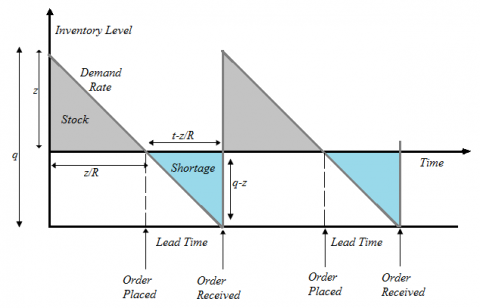Figure 2. Life cycle for economic order quantity model with a shortage

The optimization cost for the inventory system is expressed as:

$c(q)=\frac{q c_{1}}{2}+\frac{R}{q} c_{3}$                         (11)

On further simplifying, we have

$c(q)=\frac{\left(q_{p}-z\right) c_{1}}{2}+\frac{R}{q_{p}-z} c_{3}$            (12)

The total cost c(q) is optimized, when both inventories carrying costs and yearly ordering costs balanced.

$\frac{\left(q_{p}-z\right) c_{1}}{2}=\frac{R}{q_{p}-z} c_{3}$                 (13)

$q_{p}^{2}+z^{2}-2 z q_{p}-\frac{2 R c_{3}}{c_{1}}=0$            (14)

On simplifying, the performance measure for inventory stock system is derived as:

Lot size of a stock is

$z=q_{p}+\sqrt{2 R \frac{c_{3}}{c_{1}}}$                 (15)

The optimal service time to deliver the product

$t_{p}=\frac{z}{R} \pm \sqrt{\frac{2 c_{3}}{R c_{1}}}$                   (16)

Hence optimization cost per unit cycle of the time is

$c(q)=\sqrt{2 R c_{3} c_{1}}$                (17)

5.2 Graphical presentations

Results are presented in graphical form for the inventory model, discussed in this paper. Figure 3 presents the results for the effective cost of the product in the supply chain system with proportionate to the demand of the product. From this, it is observed that effective price increases with demand and tends to increases time. It is observed that the establishment cost to handle the products also affects the operative cost. Figure 4 is similar to the previous one, whereas it presents the result for order size. Here the size of the order for the products directs proportionate to the demand and increases with orders of the customers.

## 3.png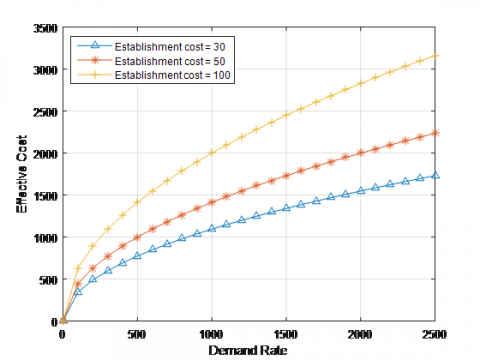Figure 3. Effective cost of the product vs. demand rate with fixed establishment cost in thousands

Figure 5 represents the stock that is available in the warehouse concerning demand. From this, it is observed that if we can provide a better service then there is a flexibility to provide the service to the customers with minimum stock. It is clear evidence that the scheduling of the products can be processed instantly with better service rate, and can be seen in the Figure 6. The optimum service rate that is required to process stock can be determined from this result with different parameters.

## 4.png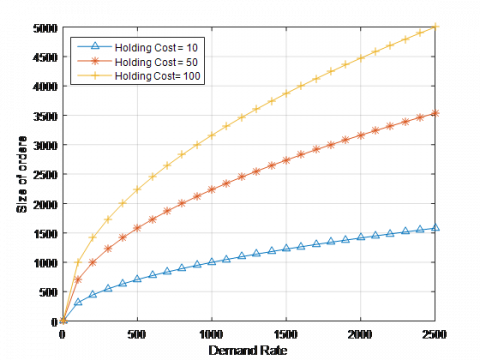Figure 4. Order size from the customers vs. demand rate with fixed holding cost

## 5.png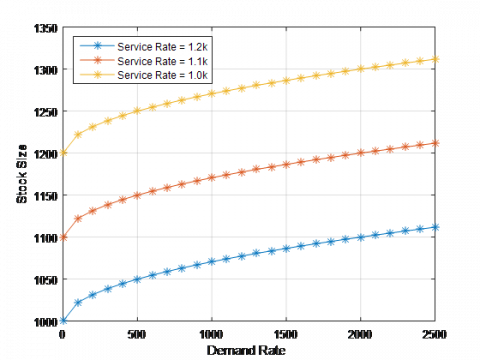Figure 5. Availability of the stock at warehouse with demand rate

## 6.png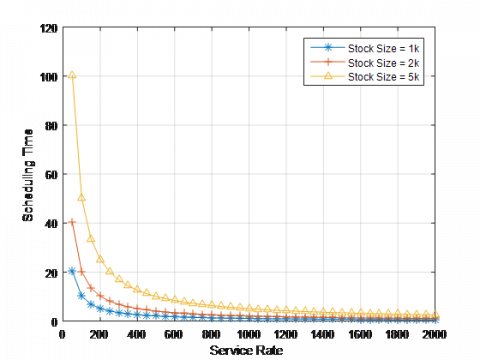Figure 6. Schedules of the orders with service rate of the products

Figure 7 presents the results for time that take schedule order with proportionate demand of the product. It exponentially decreases with the rate of the service of the product. Figure 8 illustrates that waiting to deliver products increases exponentially with increases in the orders. Here service capacities maintained with three different levels. Figure 9 presents the results for mean waiting to deliver the product to the customer with increases of the service by considering various sizes of mean arrivals. It is clear evidence that process time will not be impacted and tends to constant after with a suitable optimal service rate. Figure 10 shows that processing time for the orders increases exponentially with check out of the products. For the considerable stock size, sufficient time may not exist to deliver items to customers with bulk orders. Figure 11 shows that waiting time to deliver items increases with various shortage levels of the products.

## 7.png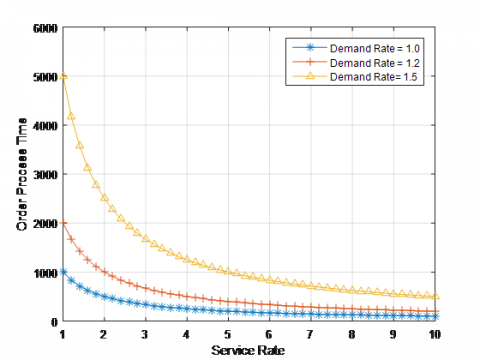Figure 7. Process time of the order with its service time

## 8.png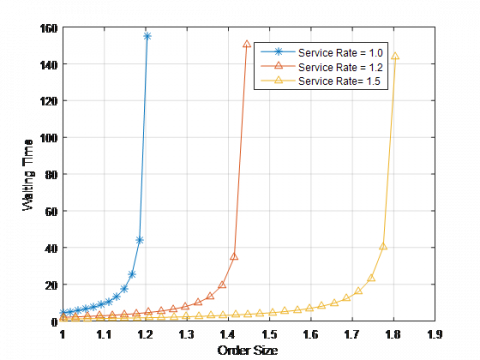Figure 8. Waiting time to deliver the product with size of the orders

## 9.png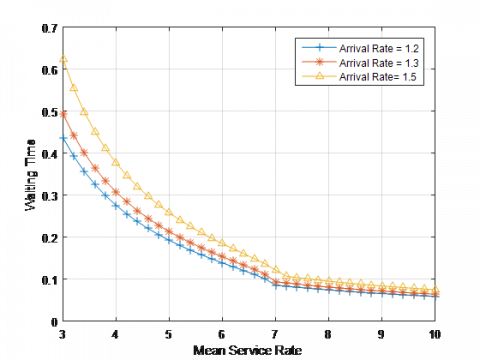Figure 9. Required time to deliver the product vs. service rate with fixed arrival orders

## 10.png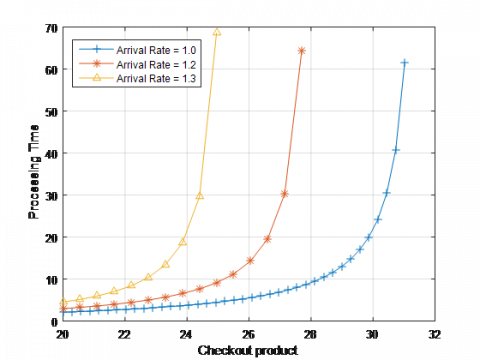Figure 10. Process time of the item with checkout of the product

## 11.png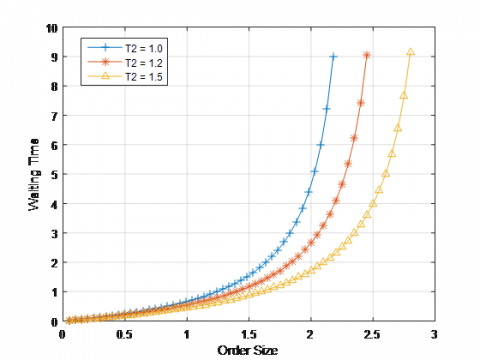Figure 11. Waiting time of the delivery of the product with order sizes

6. Conclusions

In this work, we developed a stochastic model to obtain parametric measures for a procurement policy with returns to a certain inventory system. The key use in this work is an exponential distribution to the steady-state inventory system which leads to the development of cost model.

The procurement policy is always treated as a stationary policy in a single product model, represented as M/M/1 with an infinite size waiting time queuing system. The results produced in this model can be used for reductions in total expected costs. Further, we should know how the single product inventory model solution can be incorporated into an analytic algorithm and further can be upgraded to evaluate different product stock size levels with returns of the M-echelon form of inventory control policy.

A mathematical model for inventory system is formulated with time functionality to declining product orders and marginally partial backlogging, keeping in mind the amount spent by stakeholders in the maintenance of stock and the replenishment schedule taking as decision variables. The key intention of this work is to maximize revenue per capita per unit time and to minimize expenditure cost with various investment strategies for preservation technology and optimal replenishment. Numerical results are represented in graphical forms and analyzed to the proposed model.

Acknowledgment

The authors wish to express their gratitude to the anonymous referees and the editor for their valuable guidelines and suggestions for refinement of the paper.

References

 Kennedy, W.J., Patterson, J.W., Fredendall, L.D. (2002). An overview of recent literature on spare parts inventories. International Journal of Production Economics, 76(2): 201-215. https://doi.org/10.1016/S0925-5273(01)00174-8

 Liu, L., Liu, X., Yao, D.D. (2004). Analysis and optimization of a multistage inventory-queue system. Management Science, 50(3): 365-380. https://doi.org/10.1287/mnsc.1030.0196

 Mamatha, E., Reddy, C.S., Prasad, R. (2012). Mathematical modeling of markovian queuing network with repairs, breakdown and fixed buffer. Journal on Software Engineering, 6(3): 1-21.

 Liu, L., Liu, X., Yao, D.D. (2004). Analysis and optimization of a multistage inventory-queue system. Management Science, 50(3): 365-380. https://doi.org/10.1287/mnsc.1030.0196

 Roldán, R.F., Basagoiti, R., Coelho, L.C. (2017). A survey on the inventory-routing problem with stochastic lead times and demands. Journal of Applied Logic, 24: 15-24. https://doi.org/10.1016/j.jal.2016.11.010

 Sadjadi, S.J., Makui, A., Dehghani, E., Pourmohammad, M. (2016). Applying queuing approach for a stochastic location-inventory problem with two different mean inventory considerations. Applied Mathematical Modelling, 40(1): 578-596. https://doi.org/10.1016/j.apm.2015.06.010

 Mamatha, E., Sasritha, S., Reddy, C.S. (2017). Expert system and heuristics algorithm for cloud resource scheduling. Romanian Statistical Review, 65(1): 3-18.

 Lieckens, K.T., Colen, P.J., Lambrecht, M.R. (2013). Optimization of a stochastic remanufacturing network with an exchange option. Decision Support Systems, 54(4): 1548-1557. https://doi.org/10.1016/j.dss.2012.05.057

 Li, J., Sava, A., Xie, X. (2009). An analytical approach for performance evaluation and optimization of a two-stage production-distribution system. International Journal of Production Research, 47(2): 403-414. https://doi.org/10.1080/00207540802426284

 Kao, C., Chen, S.P. (2006). A stochastic quasi-newton method for simulation response optimization. European Journal of Operational Research, 173(1): 30-46. https://doi.org/10.1016/j.ejor.2004.12.011

 Lim, E. (2012). Stochastic approximation over multidimensional discrete sets with applications to inventory systems and admission control of queueing networks. ACM Transactions on Modeling and Computer Simulation (TOMACS), 22(4): 1-19. https://doi.org/10.1145/2379810.2379812

 Diabat, A., Dehghani, E., Jabbarzadeh, A. (2017). Incorporating location and inventory decisions into a supply chain design problem with uncertain demands and lead times. Journal of Manufacturing Systems, 43: 139-149. https://doi.org/10.1016/j.jmsy.2017.02.010

 Van der Laan, E.A., Kiesmüller, G., Kuik, R., Vlachos, D., Dekker, R. (2004). Stochastic inventory control for product recovery management. In Reverse Logistics. Springer, Berlin, Heidelberg, pp. 181-220.

 Mamatha, E., Saritha, S., Reddy, C.S., Rajdurai, P. (2019). Mathematical modeling and performance anaylaysis of single server queuing system- eigenspectram. International Journal of Mathematicsin Operational Research. https://doi.org/10.1504/IJMOR.2019.10021946

 Rashid, R., Hoseini, S.F., Gholamian, M.R., Feizabadi, M. (2015). Application of queuing theory in production-inventory optimization. Journal of Industrial Engineering International, 11(4): 485-494.

 Andersson, J., Melchiors, P. (2001). A two-echelon inventory model with lost sales. International Journal of Production Economics, 69(3): 307-315. https://doi.org/10.1016/S0925-5273(00)00031-1

 Gong, Y., De Koster, R.B. (2011). A review on stochastic models and analysis of warehouse operations. Logistics Research, 3(4): 191-205.

 Hariharan, R., Zipkin, P. (1995). Customer-order information, lead times, and inventories. Management Science, 41(10): 1599-1607. https://doi.org/10.1287/mnsc.41.10.1599

 Schwarz, M., Sauer, C., Daduna, H., Kulik, R., Szekli, R. (2006). M/M/1 queueing systems with inventory. Queueing Systems, 54(1): 55-78. https://doi.org/10.1007/s11134-006-8710-5

 Wang, F.F. (2012). A generic modeling framework for queueing-inventory systems with a single removable server. Journal of the Chinese Institute of Industrial Engineers, 29(1): 1-15. https://doi.org/10.1080/10170669.2011.653994

 Giri, B.C., Bardhan, S. (2016). Coordinating a two-echelon supply chain with price and inventory level dependent demand, time dependent holding cost, and partial backlogging. International Journal of Mathematics in Operational Research, 8(4): 406-423. https://doi.org/10.1504/IJMOR.2016.076782

 Pankratov, E.L. (2019). On optimization of inventory management of an industrial enterprise. On analytical approach for prognosis of processes. Advances in Modelling and Analysis A, 56(1): 26-29. https://doi.org/10.18280/ama_a.560105

 Mamatha, E., Saritha, S., Reddy, C.S. (2016). Stochastic scheduling algorithm for distributed cloud networks using heuristic approach. International Journal of Advanced Networking and Applications, 8(1): 3009.

 Ching, W.K., Yuen, W.O., Loh, A.W. (2003). An inventory model with returns and lateral transshipments. Journal of the Operational Research Society, 54(6): 636-641. https://doi.org/10.1057/palgrave.jors.2601521

 Tan, J., Wang, Z.G., Jiang, G.Q. (2018). Modelling and simulation of the balance of supply chain ecosystem. Journal Européen des Systèmes Automatisés, 51(4-6): 273-281. https://doi.org/10.3166/JESA.51.273-281